﻿ How to Draw 3D Charts with Matplotlib – BMC Software | Blogs
Machine Learning & Big Data Blog

# How to Draw 3D Charts with MatplotlibIn this article, I’ll show how to draw three-dimensional charts in Matplotlib.

To plot charts in Matplotlib, you need to use a Zeppelin or Jupyter notebook (or another graphical environment). Your other option is to save your charts to a graphics file in order to display them later. This is particularly useful if you’re executing a long-running program that takes too many minutes or hours to run in an interactive notebook, such as a machine learning model.

## Introduction to 2D charts

I’ll start with a very easy explanation of the basic concepts. The flat surface of a chart is also known as the cartesian plane. You remember from high school that each point (x,y) is a point on this x-y flat surface.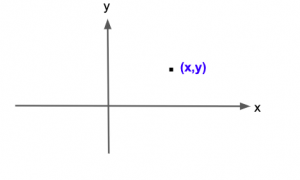The plot of a line with a 45-degree angle, for example, is f(x)=y=x. We usually write y=f(x) to mean x is a function of y.

Let’s plot y = sin(x) for the familiar curve that ranges between 1 and -1. Here we go.

We first fill an array of enough data points to make a smooth chart. In particular we set them 0.1 apart and range from -5 to 5:

`x = np.arange(-5,5,0.1)`

The rest of the code is simple. bo means blue circle.

```import matplotlib.pyplot as plt
fig = plt.figure(figsize=(10,10))

x = np.arange(-5,5,0.1)
y = np.sin(x)

plt.plot(x,y,'bo')
```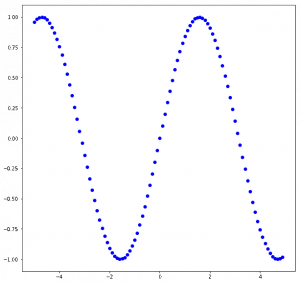Now, let’s add a third dimension to our first chart.

## 3D charting in Matplotlib

First, a caveat: People don’t use 3D charts often, mostly because readers have a difficult time understanding the charts. For example, it’s easy to read a 2D time-series chart, with time on the x-axis and y on the vertical axis. But if we add a third z-point, it’s floating in space, often resembling a blob and making the meaning hard to grasp.

Here, I will do something simple—place our flat sine curve in 2D space. This is technically called a hyperplane, since it has no dimension in the 3rd dimension.

In 3D space, each coordinate is given as (x,y,z). In calculus class, you’re used to seeing the coordinate system expressed like this: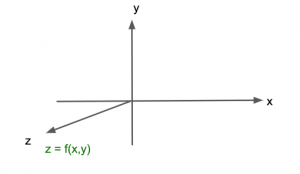The point z is a function of x and y. In charting, the principle is the same except these arrows (axes) are moved to the middle of the box, enclosing the chart space. The tick marks (e.g., -2, -1, 0, 1, 2) are drawn on the box that encloses those axes, which has not been moved to the origin, i.e. (x=0,y=0,z=0).

The direction of the z arrow can be up, out, or across. It’s just a matter of picking which orientation you find easiest to understand.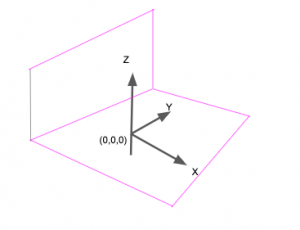## 3D chart example

Here is a sample chart. We will draw the same sine curve as we drew on the flat cartesian plane. But here we will set z to the constant value 0. Thus, all points are on the hyperplane (x,y,0).

This creates the illusion of 3D space, making our graph appear to float in space. That’s the whole point of making 3D charts: to add one more axis to the visual presentation. Of course, humans cannot see any dimension beyond three, certainly not four dimensions.

Since this chart is a curve we can just use plot(), since we don’t have pointing jumping all over the place. (If we did we would probably use scatter()).

We first set out canvas to 3D. And this method gives us access to the axes object:

```from mpl_toolkits.mplot3d import Axes3D
ax = fig.gca(projection='3d')
```

Here we will set z, written as zs, to 0. So, this is the plot of (x,y,0). zdir means which direction to 3D. The default size might otherwise be too small.

Then we can use the plot() method:

```fig = plt.figure(figsize=(10,10))
ax.plot(x,y,'bo', zs=0, zdir='y')
```

## The code

Here is the complete code. The rest of the code we use to show the origin, point (0,0,0), at the center so it’s easier to see.

```import matplotlib.pyplot as plt
from mpl_toolkits.mplot3d import Axes3D
import numpy as np

x = np.arange(-5,5,0.1)
y = np.sin(x)
fig = plt.figure(figsize=(10,10))
ax = fig.gca(projection='3d')
ax.plot(x,y,'bo', zs=0, zdir='y')

origin = [0,0,0]
ax.text(origin,origin,origin,"origin",size=20)

fig.show()
```

Of course, data scientists are not plotting functions most of the time. They are plotting arrays. But using functions is the easiest way to illustrate this, as most programmers are familiar with those.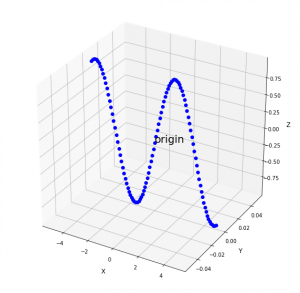This e-book teaches machine learning in the simplest way possible. This book is for managers, programmers, directors – and anyone else who wants to learn machine learning. We start with very basic stats and algebra and build upon that.

These postings are my own and do not necessarily represent BMC's position, strategies, or opinion.

See an error or have a suggestion? Please let us know by emailing blogs@bmc.com.

### BMC Bring the A-Game

From core to cloud to edge, BMC delivers the software and services that enable nearly 10,000 global customers, including 84% of the Forbes Global 100, to thrive in their ongoing evolution to an Autonomous Digital Enterprise.